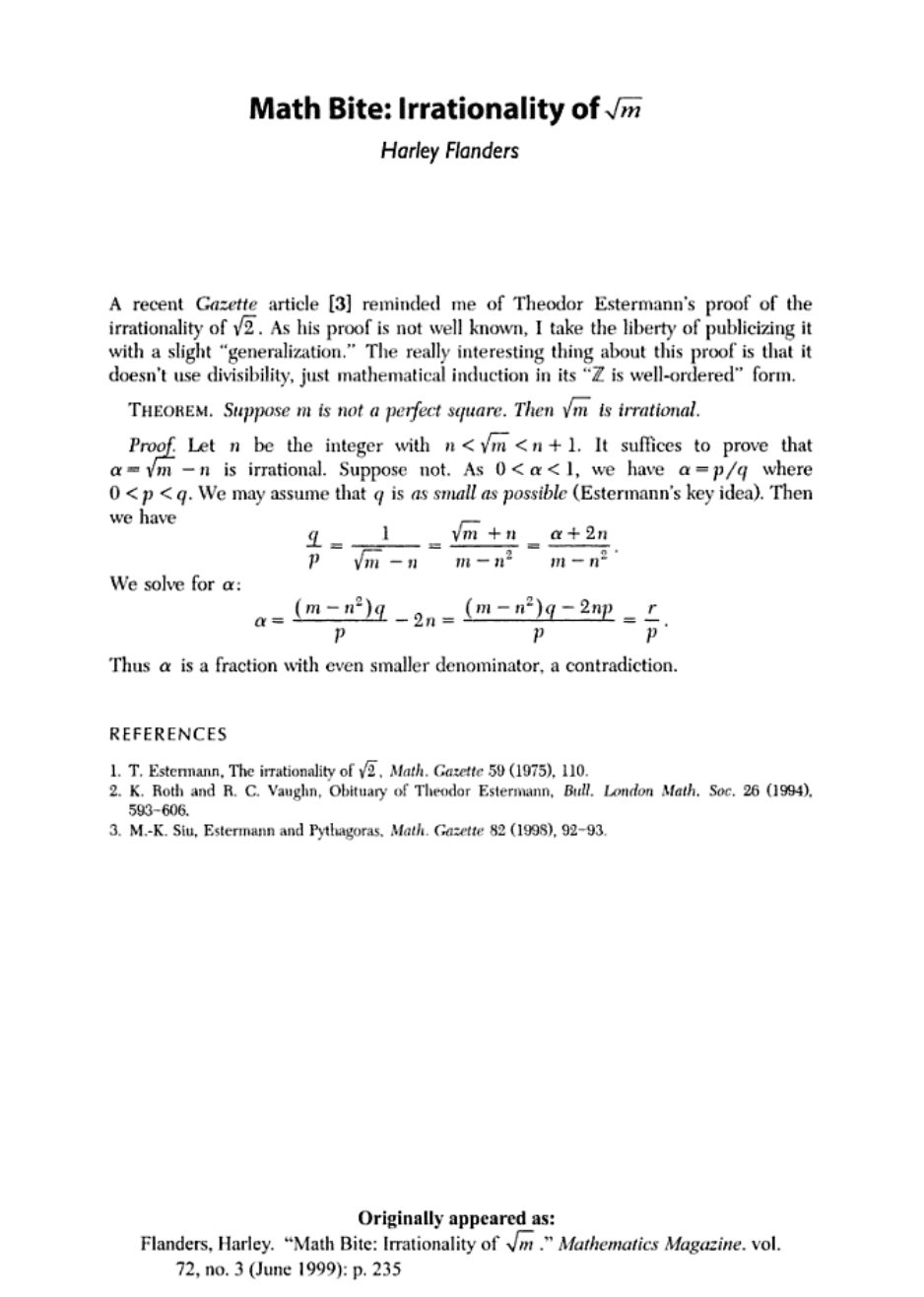Theodor Estermann proved the irrationality of $\sqrt2$ without rely...
Exactly why is it sufficient to prove sqrt(m) - n is irrational? Th...
Note that $q$ is by assumption the smallest integer we can use as a...
In fact it is even possible to generalize: for positive integers $m...Exactly why is it sufficient to prove sqrt(m) - n is irrational? This stopped me cold. rational + irrational = irrational (if rational + irrational = rational we would get irrational = rational - rational, but rationals are closed under addition and subtraction). Hence (sqrt(m) - n) + n = sqrt(m) is irrational (irrational + rational = irrational) This sort of argument is common for properties that are closed under some operation (like rationality with addition). Theodor Estermann proved the irrationality of$\sqrt2$without relying on the prime factorization of$m$. He claimed that his proof was even easier than [Pythagoras' proof](https://en.wikipedia.org/wiki/Square_root_of_2#Pythagorean_theorem_proof). Estermann started by defining a set$S$of all natural numbers$n$such that$n\sqrt2$is an integer. If the set$S$was not empty, it would have a least element$k$, for instance. Now if we consider: $$(\sqrt2 -1)k\sqrt 2=2k -k\sqrt2$$ Since$k\in S$, both$(\sqrt2 -1)k$and$2k -k\sqrt2$are naturals. So by definition$(\sqrt2 -1)k \in S$. However$(\sqrt2 -1)k < k$, contradicting the initial assumption that k is the least element of S. This way we conclude that$S$is empty and$\sqrt2$must be irrational. Flanders generalizes the proof of Estermann by replacing 2 by$m$and replacing 1 by the greatest integer less than$\sqrt m$. In fact it is even possible to generalize: for positive integers$m$and$k$,$^{k}\sqrt m$is rational only when$m$is a perfect$k^{th}$power. To prove this let us suppose that$^{k}\sqrt m=\frac{a}{b}$where$a,b>0$and are integers and the Greatest Common Divisor between$a$and$b$is 1. Now $$^{k}\sqrt m=\frac{a}{b} \equiv m = \frac{a^k}{b^k} \equiv mb^k = a^k$$ If$b$has a prime factor$c$that does not divide$a$, then$c$does not divide any positive power of$a$. If$b>1$,$a^k$can be written in terms of powers of$b$($a^k=mb^k$) and consequently contradicts the initial assumption, since$a$and$b$don't have any common divisors apart from 1. The only option we have is$b=1$and in that case$m=a^k$is a perfect power. You can remove the first$k$digits in the decimal expansion of any irrational number without changing its irrationality. So therefore a number is irrational iff its fractional part is also irrational. Since$n < \sqrt{m} < n+1$,$\sqrt{m} - n$is precisely this fractional part. Note that$q$is by assumption the smallest integer we can use as a denominator in$\alpha$. Since$m,n,q$and$p$are integers$r=(m-n^2)q-2np$is also an integer and so we were able to rewrite$\alpha$as a quotient of 2 integers but this time with$p$in the denominator. As$p<q$we have reached a contradiction and$\sqrt m\$ must be irrational.